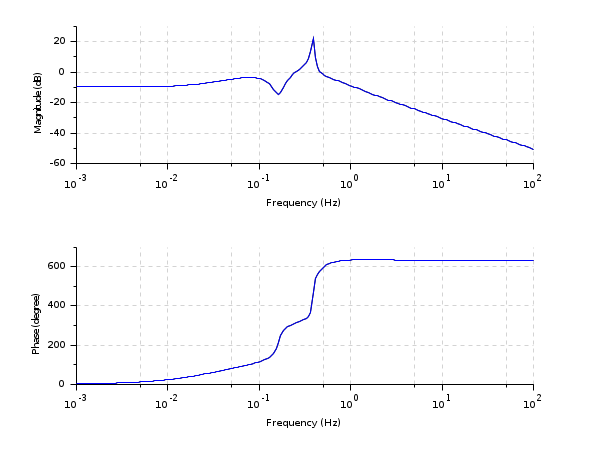Change language to:
English - Français - 日本語 - Русский

See the recommended documentation of this function

Ajuda do Scilab >> CACSD > Linear System Representation > frep2tf

# frep2tf

transfer function realization from frequency response

### Syntax

`[h [,err]]=frep2tf(frq,repf,dg [,dom,tols,weight])`

### Arguments

frq

vector of frequencies in Hz.

repf

vector of frequency response

dg

degree of linear system

dom

time domain (`'c'` or `'d'` or `dt`)

tols

a vector of size 3 giving the relative and absolute tolerance and the maximum number of iterations (default values are `rtol=1.e-2; atol=1.e-4, N=10`).

weight

vector of weights on frequencies

h

SISO transfer function

err

error (for example if `dom='c'` `sum(abs(h(2i*pi*frq) - rep)^2)/size(frq,*)`)

### Description

Frequency response to transfer function conversion. The order of `h` is a priori given in `dg` which must be provided. The following linear system is solved in the least square sense.

`weight(k)*(n( phi_k) - d(phi_k)*rep_k)=0, k=1,..,n`

where `phi_k= 2*%i*%pi*frq` when `dom='c'` and `phi_k=exp(2*%i*%pi*dom*frq` if not. If the `weight` vector is not given a default penalization is used (when `dom='c'`).

A stable and minimum phase system can be obtained by using function `factors`.

### Examples

```s=poly(0,'s');
h=syslin('c',(s-1)/(s^3+5*s+20))
frq=0:0.05:3;
repf=repfreq(h,frq);
clean(frep2tf(frq,repf,3))```
```Sys=ssrand(1,1,10);
frq=logspace(-3,2,200);
[frq,rep]=repfreq(Sys,frq);  //Frequency response of Sys
[Sys2,err]=frep2tf(frq,rep,10);
Sys2=clean(Sys2) //Sys2 obtained from freq. resp of Sys

[frq,rep2]=repfreq(Sys2,frq); //Frequency response of Sys2
clf();
bode(frq,[rep;rep2])   //Responses of Sys and Sys2
[gsort(spec(Sys('A'))), gsort(roots(Sys2('den')))] //poles``````dom = 1/1000; // Sampling time
z = poly(0,'z');
h = syslin(dom,(z^2+0.5)/(z^3+0.1*z^2-0.5*z+0.08))
frq = (0:0.01:0.5)/dom;
repf = repfreq(h,frq);
[Sys2,err] = frep2tf(frq,repf,3,dom);
[frq,rep2] = repfreq(Sys2,frq); //Frequency response of Sys2
clf();
plot2d(frq, abs([repf;rep2])')```